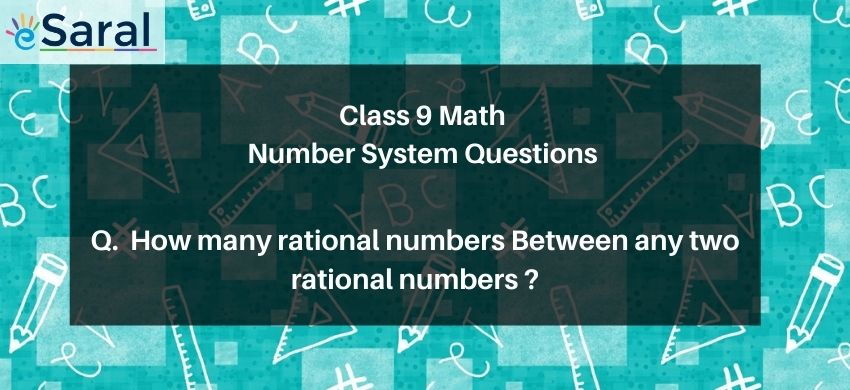Most Affordable JEE | NEET | 8,9,10 Preparation by Kota's Top IITian Doctor Faculties

# Between any two rational numbers, there are infinitely many rational numbers# Question : Between any two rational numbers

1. there is no rational number
2. there is exactly one rational number
3. there are infinitely many rational numbers
4. there are only rational numbers and no irrational numbers
Solution :
The correct option is 3. there are infinitely many rational numbers
Recall that to find a rational number between r and s, you can add
$\mathrm{r}$ and $\mathrm{s}$ and divide the sum by 2 , that is $\frac{\mathrm{r}+\mathrm{s}}{2}$ lies between $\mathrm{r}$ and $\mathrm{s}$.
For example, $\frac{5}{2}$ is a number between 2 and 3
We can proceed in this manner to find many more rational numbers between 2 and 3.
Hence, we can conclude that there are infinitely many rational numbers between any two given rational numbers.